Learn all Concepts of Linear Equations Class 9 (with VIDEOS). Check - Linear Equations in 2 Variables Class 9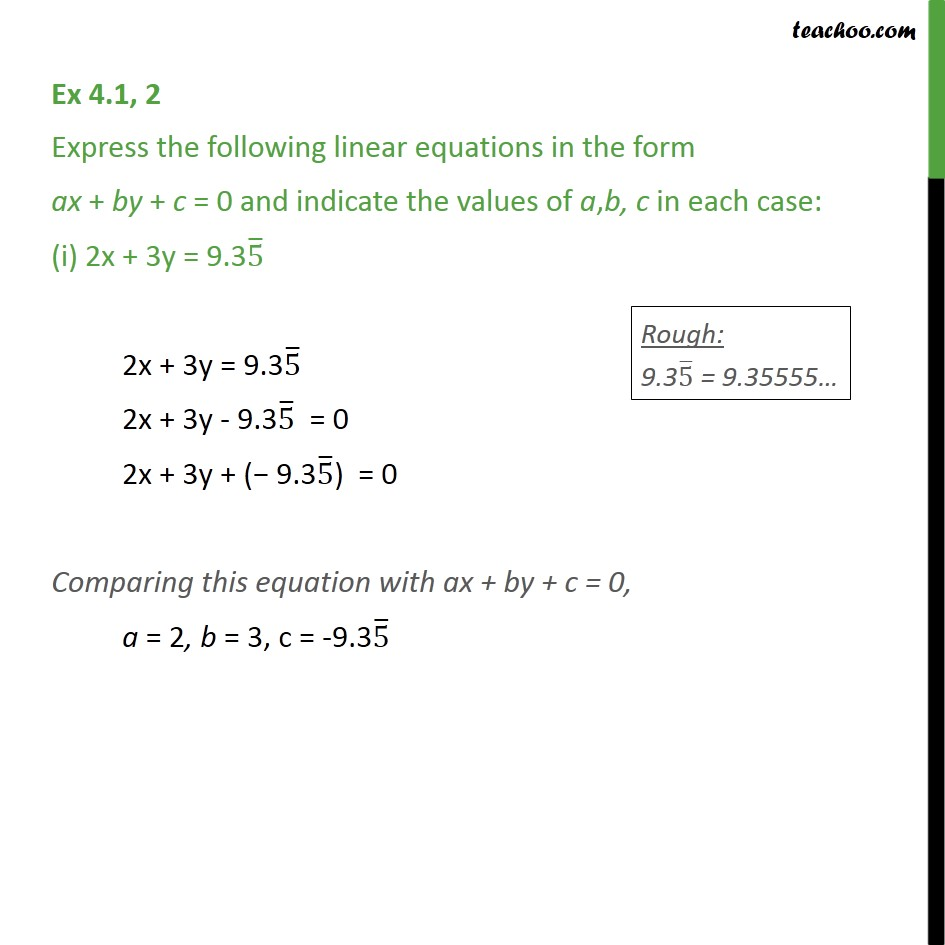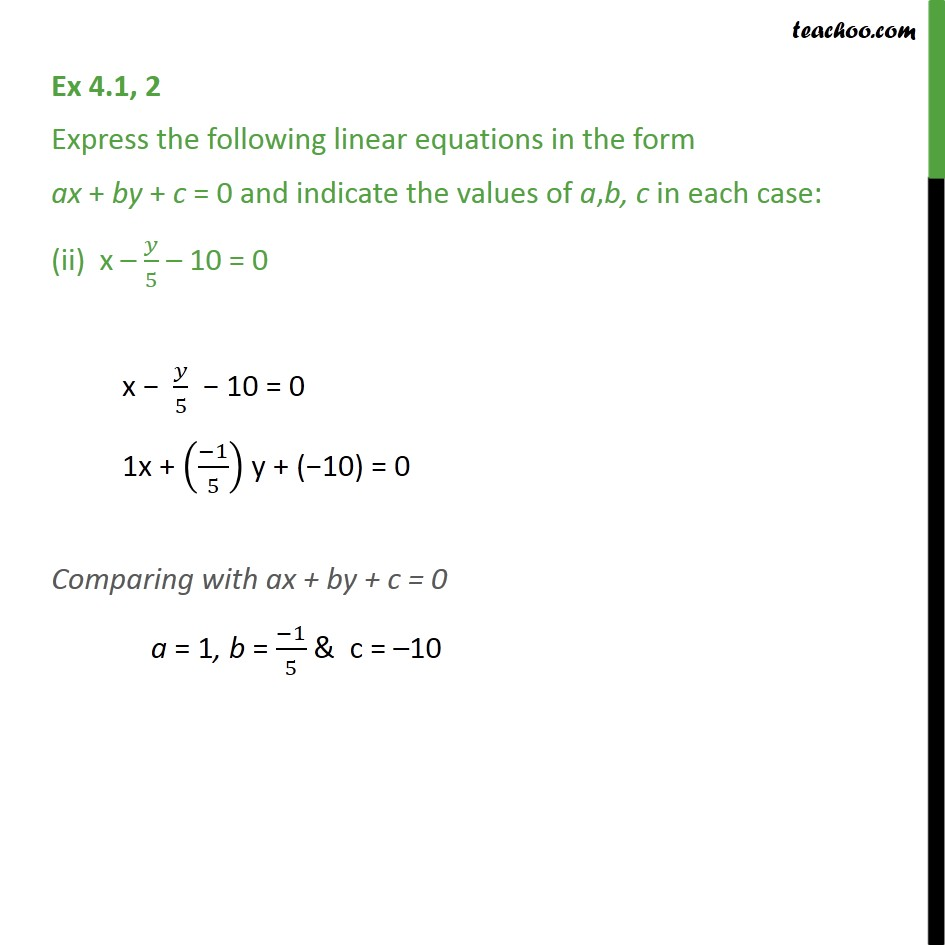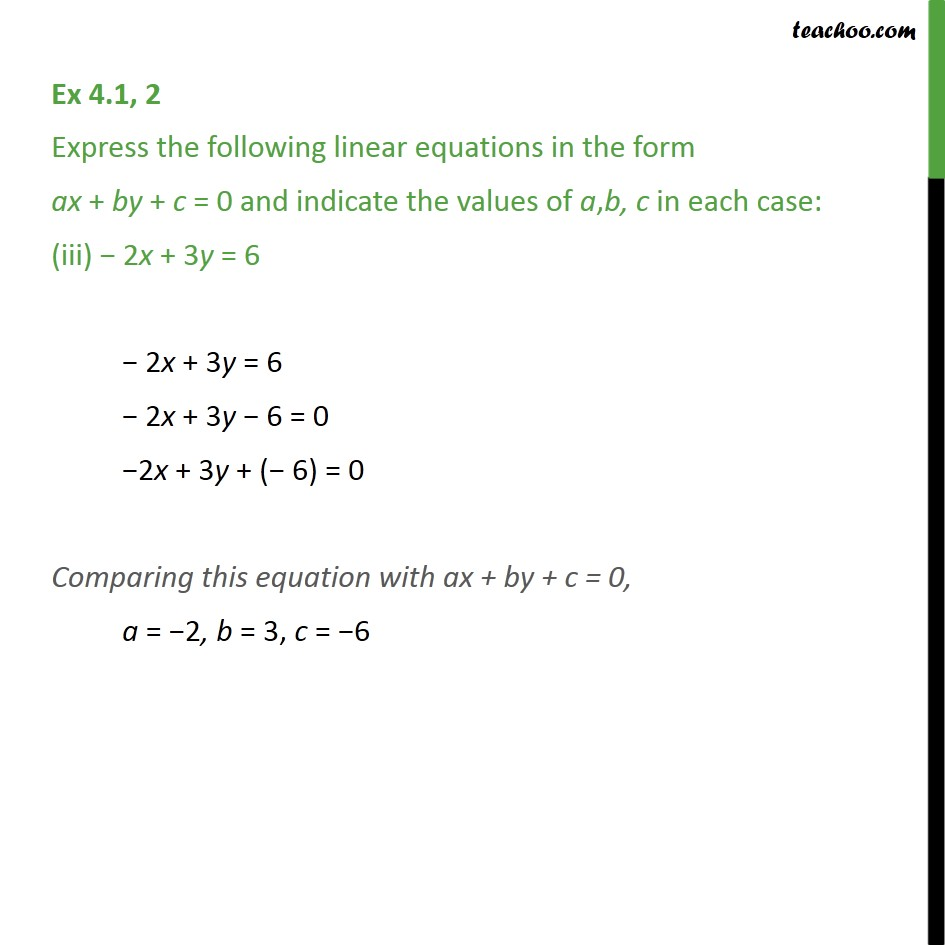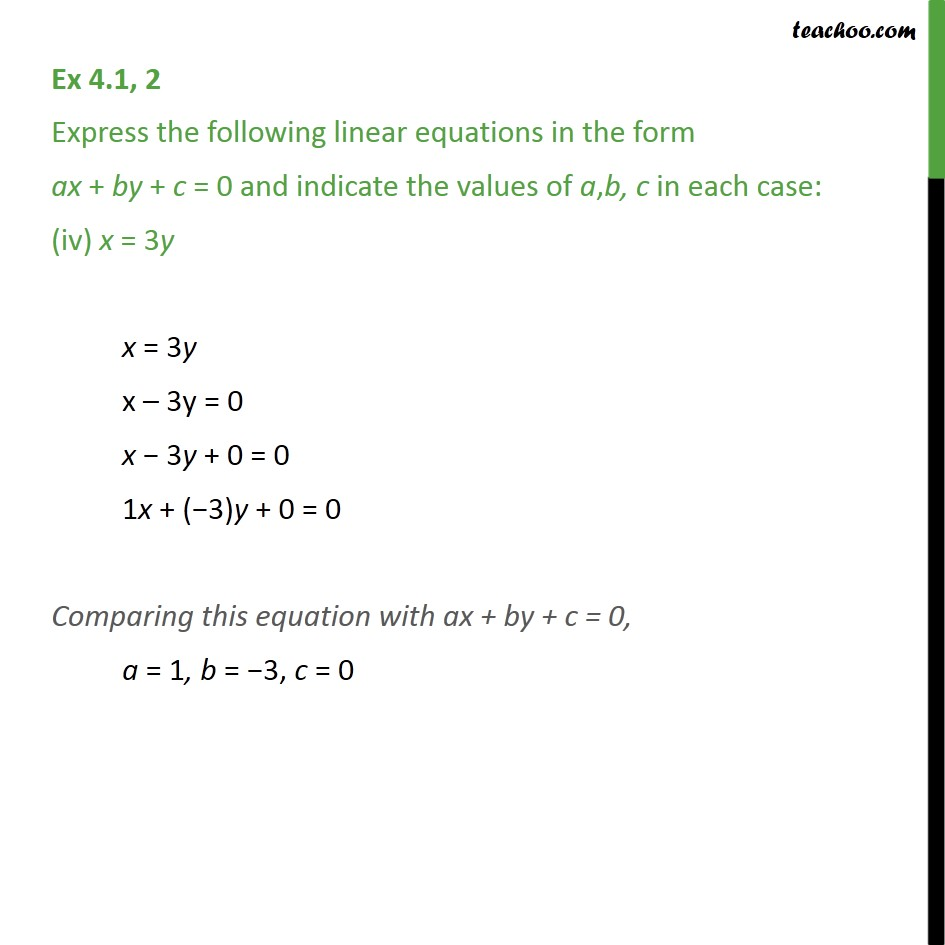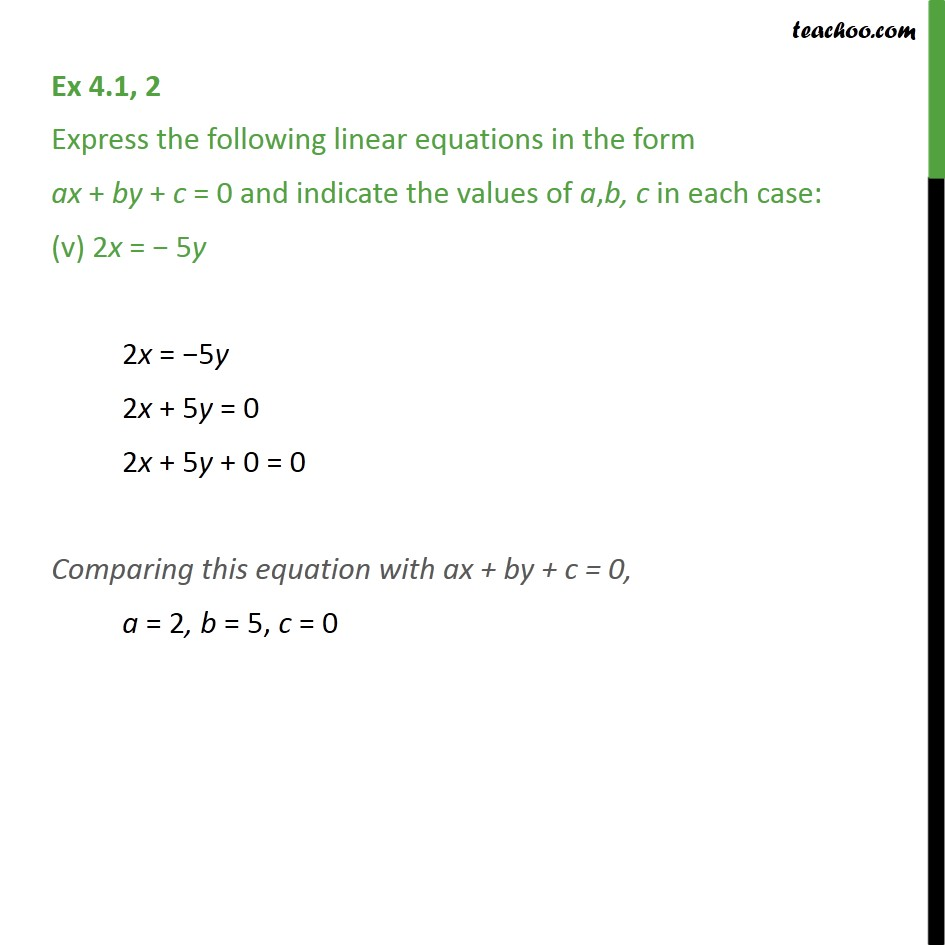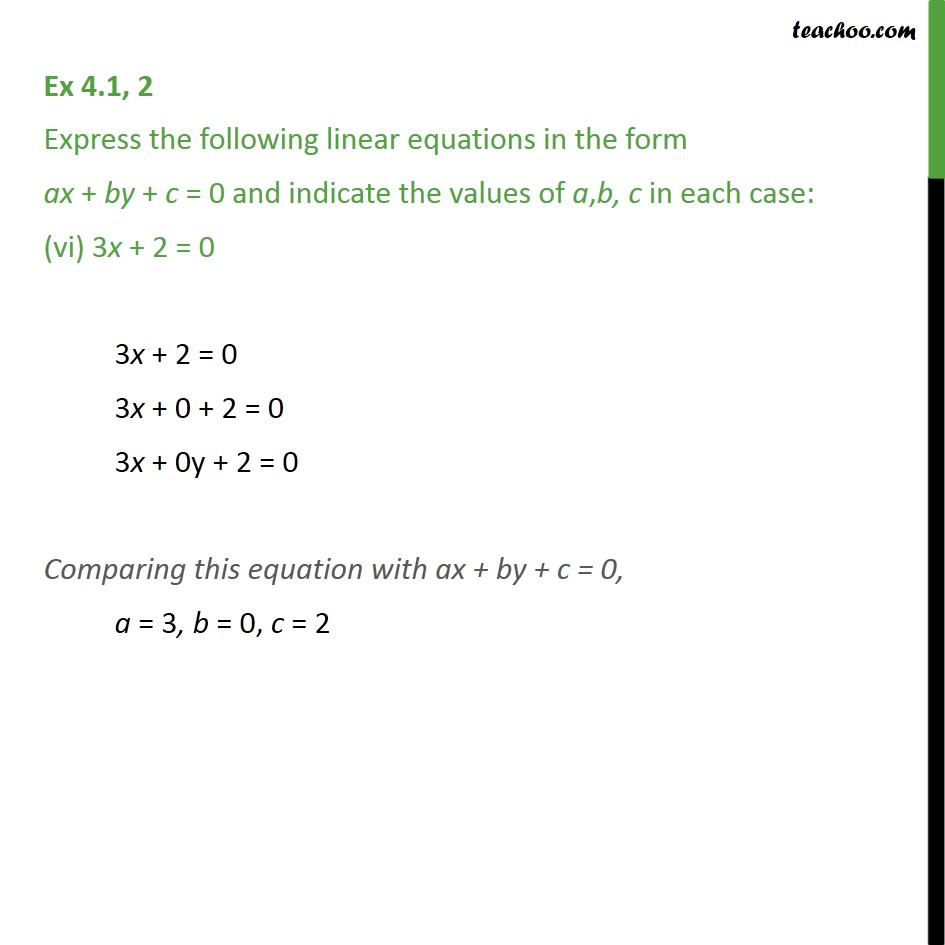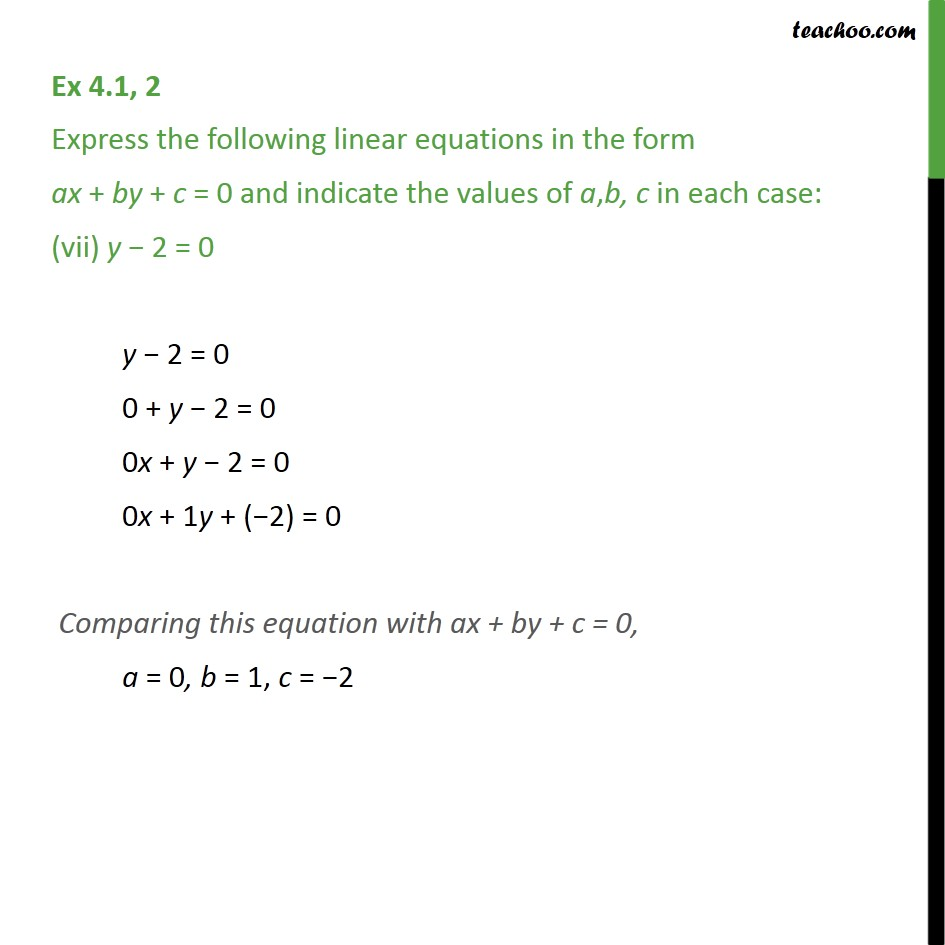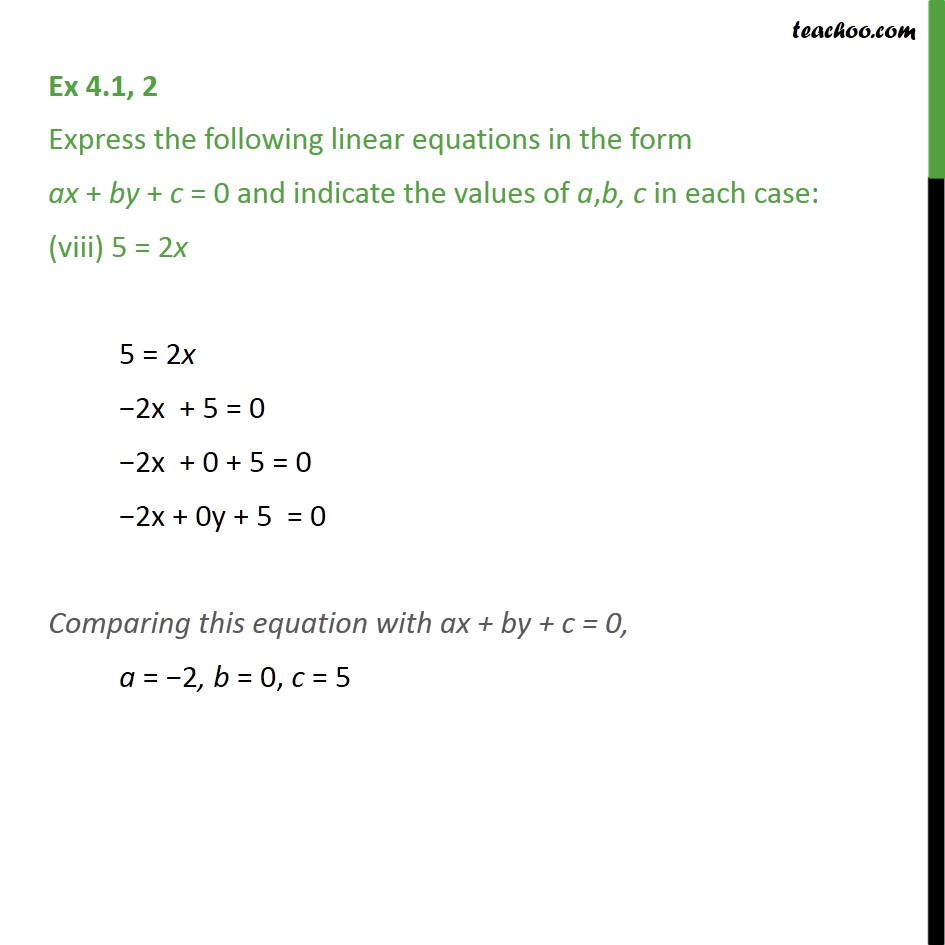1. Chapter 4 Class 9 Linear Equations in Two Variables
2. Serial order wise
3. Ex 4.1

Transcript

Ex 4.1, 2 Express the following linear equations in the form ax + by + c = 0 and indicate the values of a,b, c in each case: (i) 2x + 3y = 9.35 ̅ 2x + 3y = 9.35 ̅ 2x + 3y - 9.35 ̅ = 0 2x + 3y + (− 9.35 ̅) = 0 Comparing this equation with ax + by + c = 0, a = 2, b = 3, c = -9.35 ̅ Ex 4.1, 2 Express the following linear equations in the form ax + by + c = 0 and indicate the values of a,b, c in each case: (ii) x – 𝑦/5 – 10 = 0 x − 𝑦/5 − 10 = 0 1x + ((−1)/5) y + (−10) = 0 Comparing with ax + by + c = 0 a = 1, b = (−1)/5 & c = –10 Ex 4.1, 2 Express the following linear equations in the form ax + by + c = 0 and indicate the values of a,b, c in each case: (iii) − 2x + 3y = 6 − 2x + 3y = 6 − 2x + 3y − 6 = 0 −2x + 3y + (− 6) = 0 Comparing this equation with ax + by + c = 0, a = −2, b = 3, c = −6 Ex 4.1, 2 Express the following linear equations in the form ax + by + c = 0 and indicate the values of a,b, c in each case: (iv) x = 3y x = 3y x – 3y = 0 x − 3y + 0 = 0 1x + (−3)y + 0 = 0 Comparing this equation with ax + by + c = 0, a = 1, b = −3, c = 0 Ex 4.1, 2 Express the following linear equations in the form ax + by + c = 0 and indicate the values of a,b, c in each case: (v) 2x = − 5y 2x = −5y 2x + 5y = 0 2x + 5y + 0 = 0 Comparing this equation with ax + by + c = 0, a = 2, b = 5, c = 0 Ex 4.1, 2 Express the following linear equations in the form ax + by + c = 0 and indicate the values of a,b, c in each case: (vi) 3x + 2 = 0 3x + 2 = 0 3x + 0 + 2 = 0 3x + 0y + 2 = 0 Comparing this equation with ax + by + c = 0, a = 3, b = 0, c = 2 Ex 4.1, 2 Express the following linear equations in the form ax + by + c = 0 and indicate the values of a,b, c in each case: (vii) y − 2 = 0 y − 2 = 0 0 + y − 2 = 0 0x + y − 2 = 0 0x + 1y + (−2) = 0 Comparing this equation with ax + by + c = 0, a = 0, b = 1, c = −2 Ex 4.1, 2 Express the following linear equations in the form ax + by + c = 0 and indicate the values of a,b, c in each case: (viii) 5 = 2x 5 = 2x −2x + 5 = 0 −2x + 0 + 5 = 0 −2x + 0y + 5 = 0 Comparing this equation with ax + by + c = 0, a = −2, b = 0, c = 5

Ex 4.1